# Surface Area Calculator

Find the surface area of a shape by entering the dimensions and selecting a unit of measurement.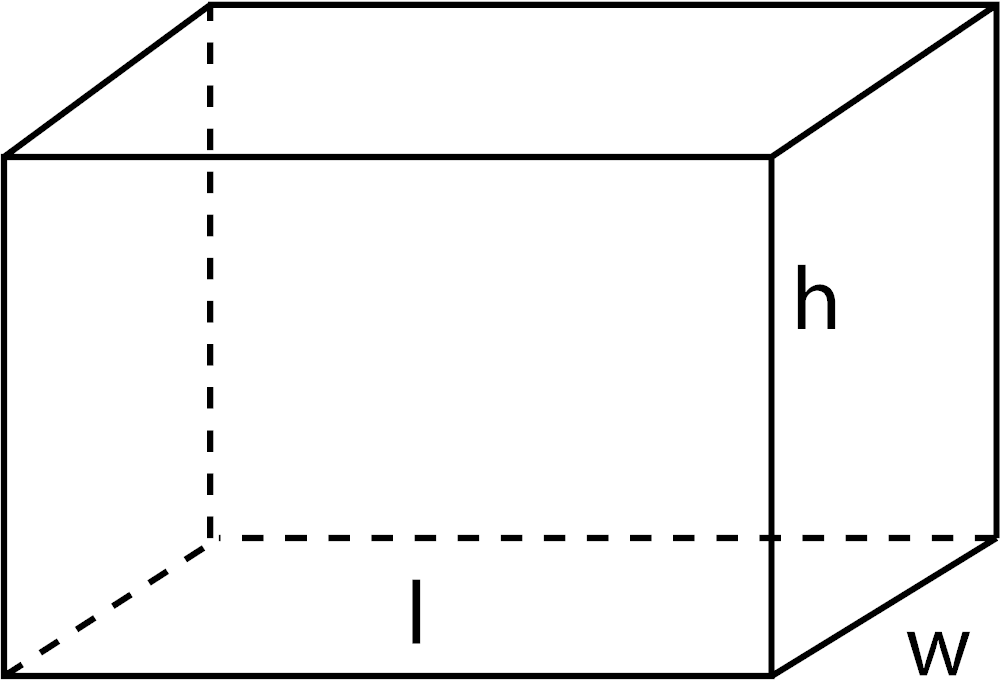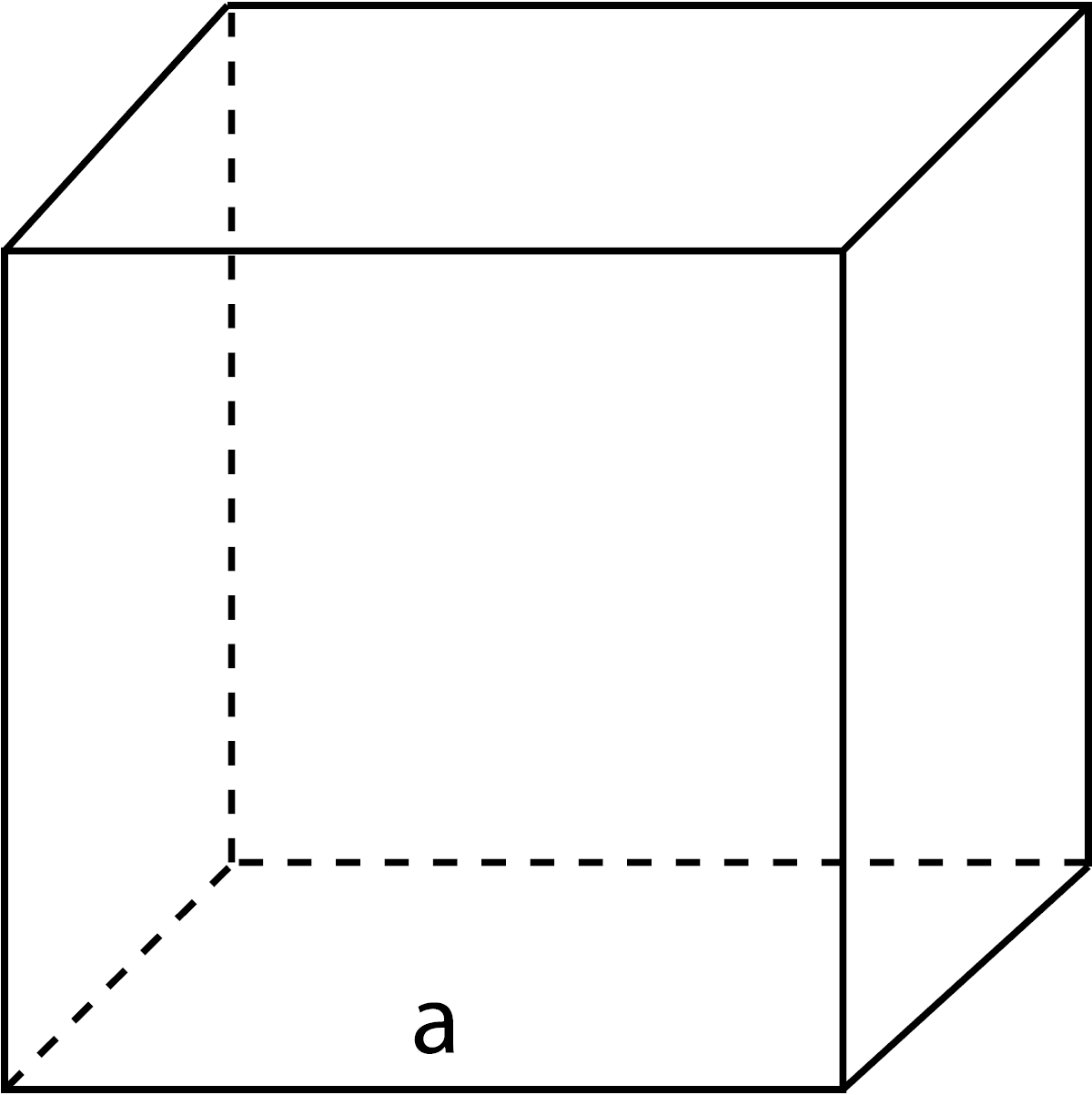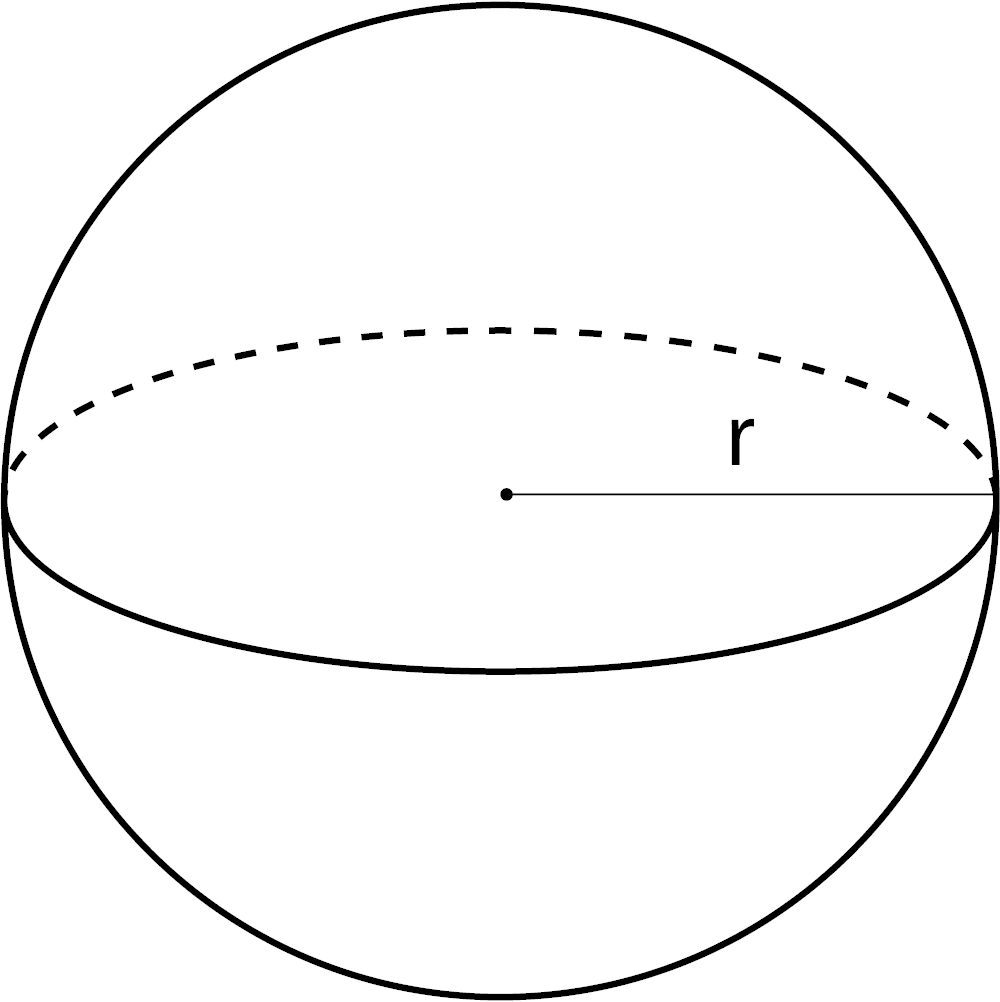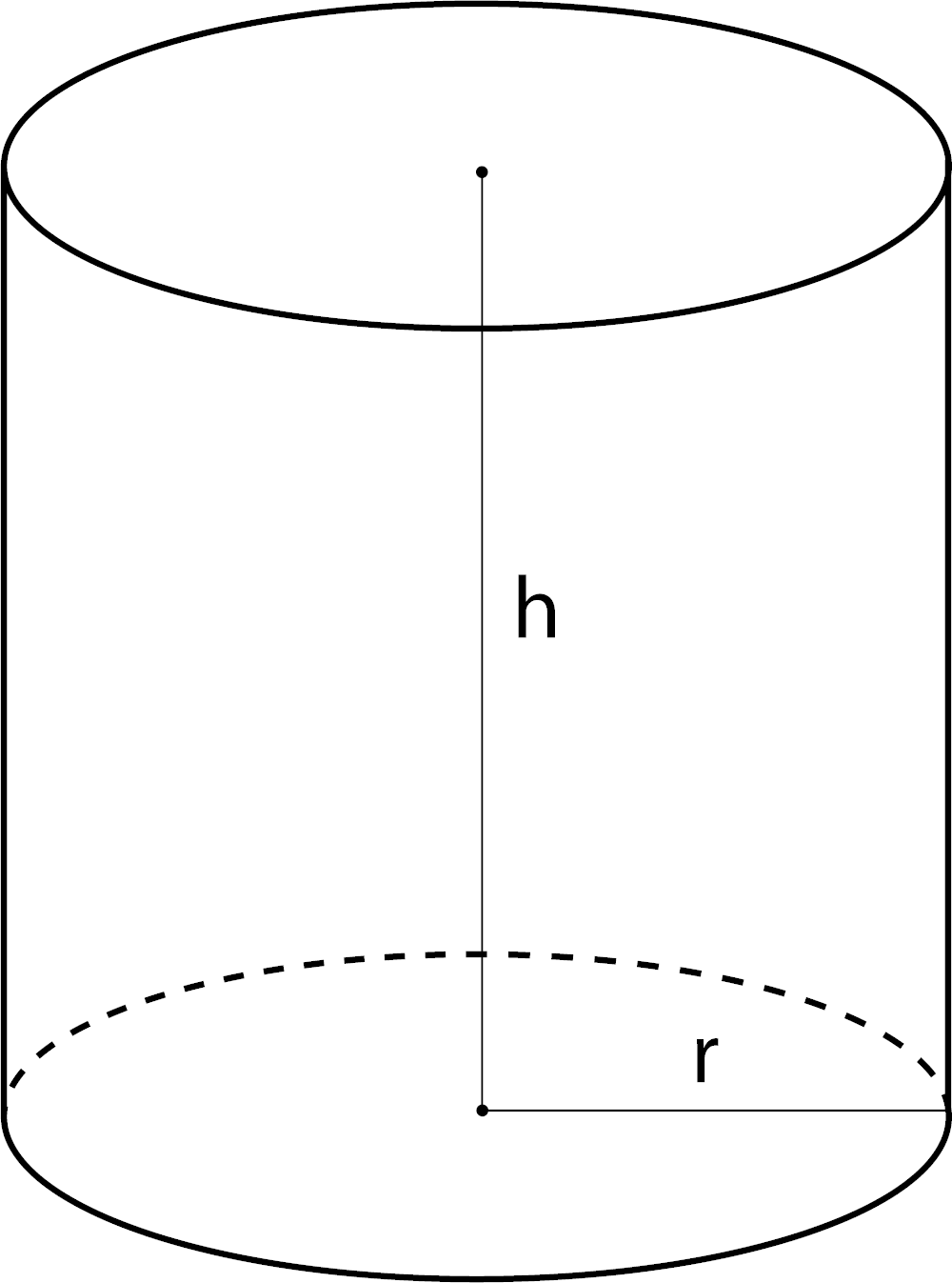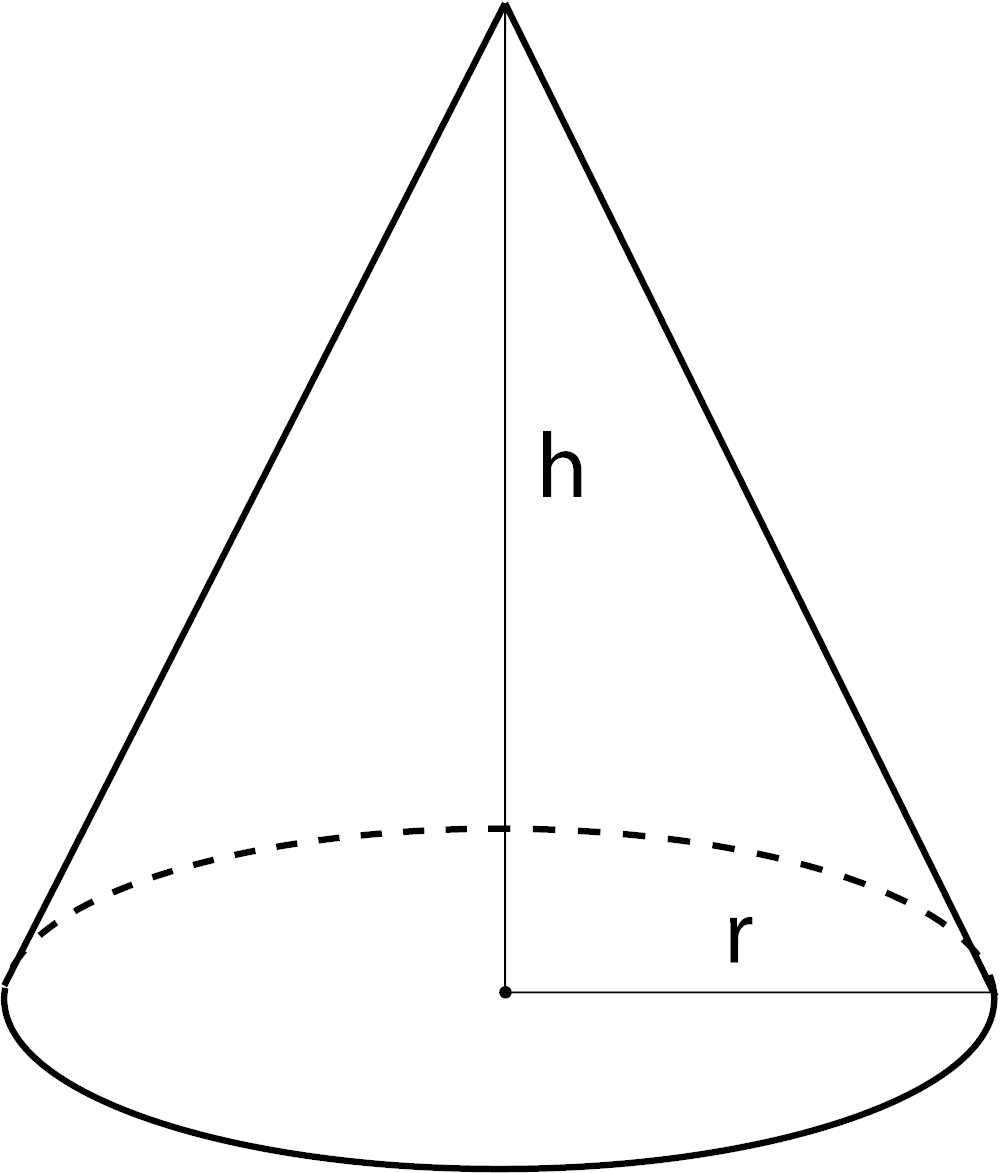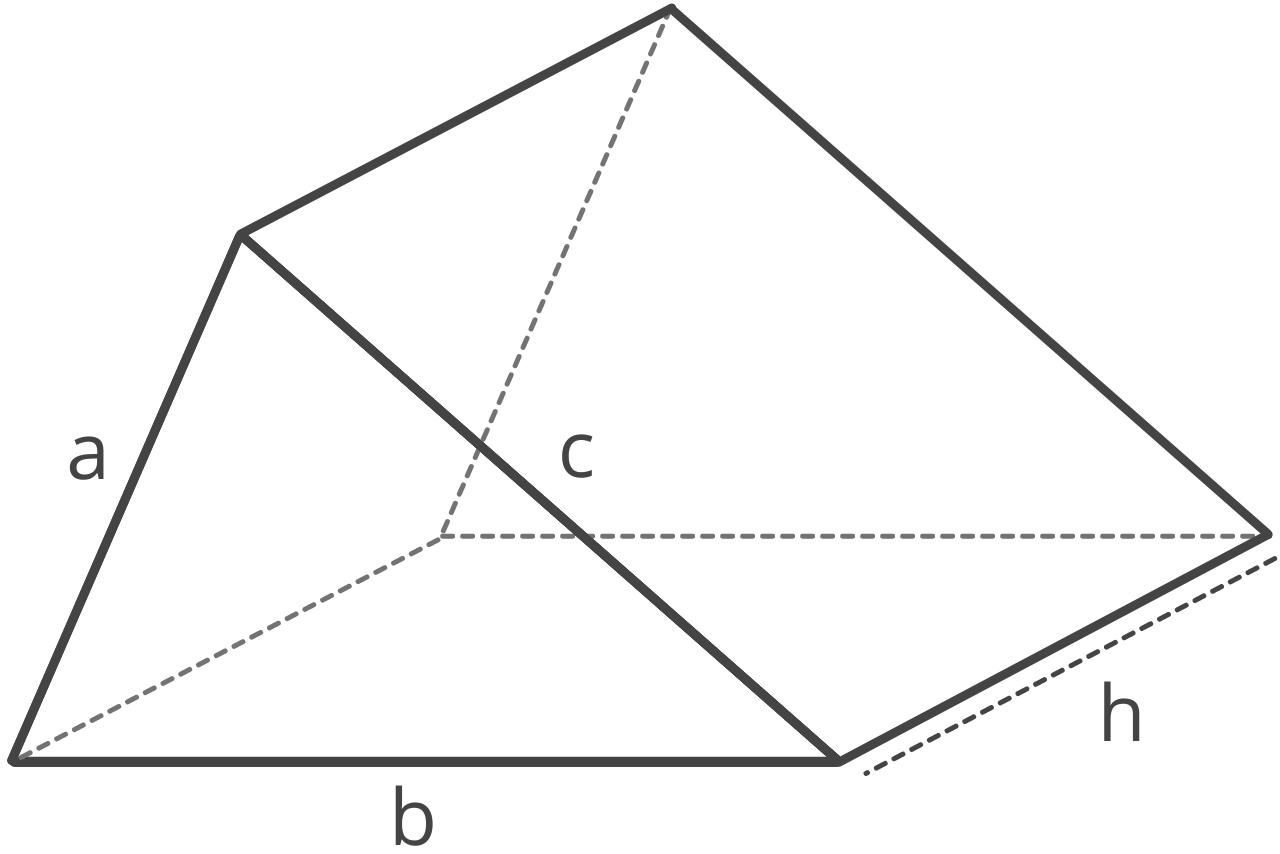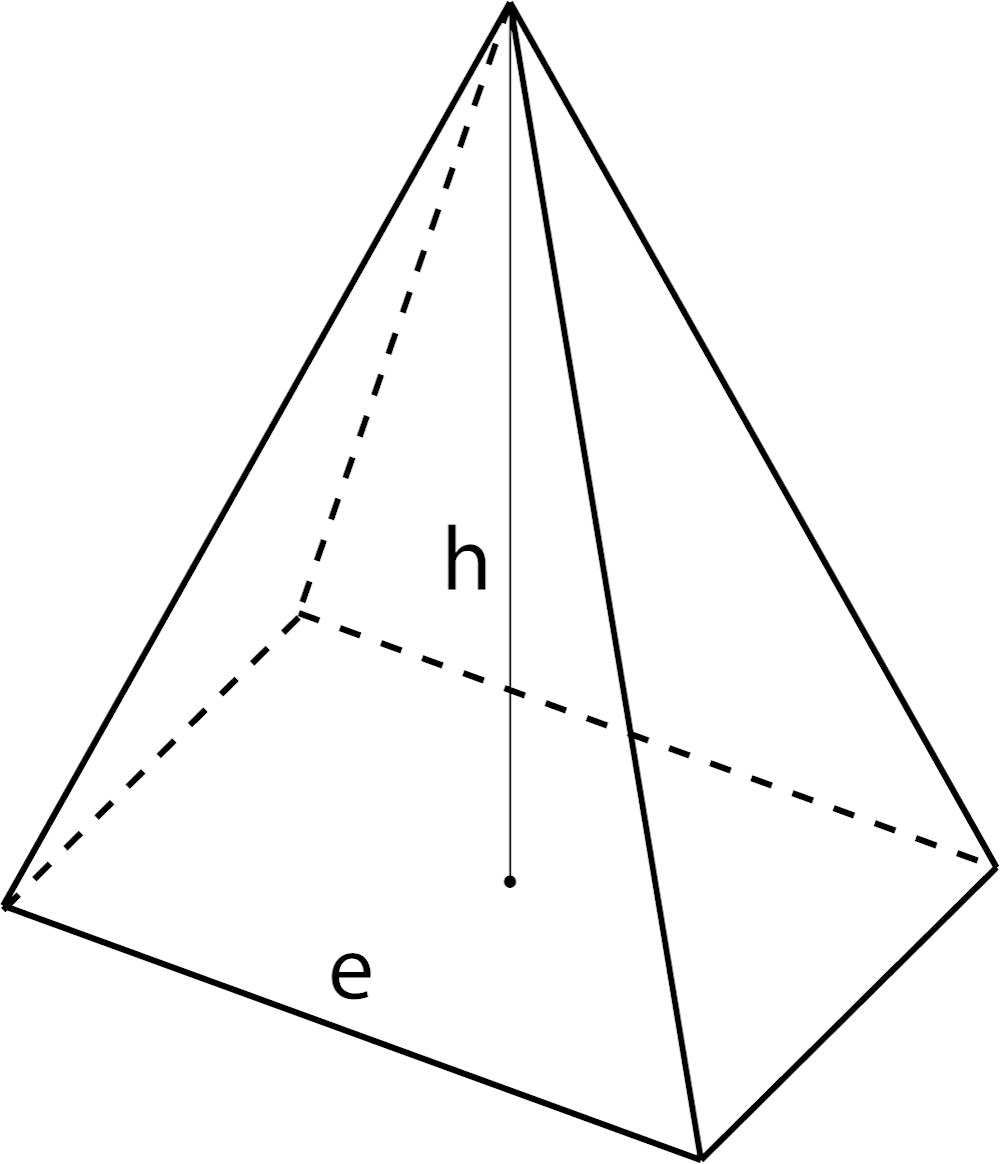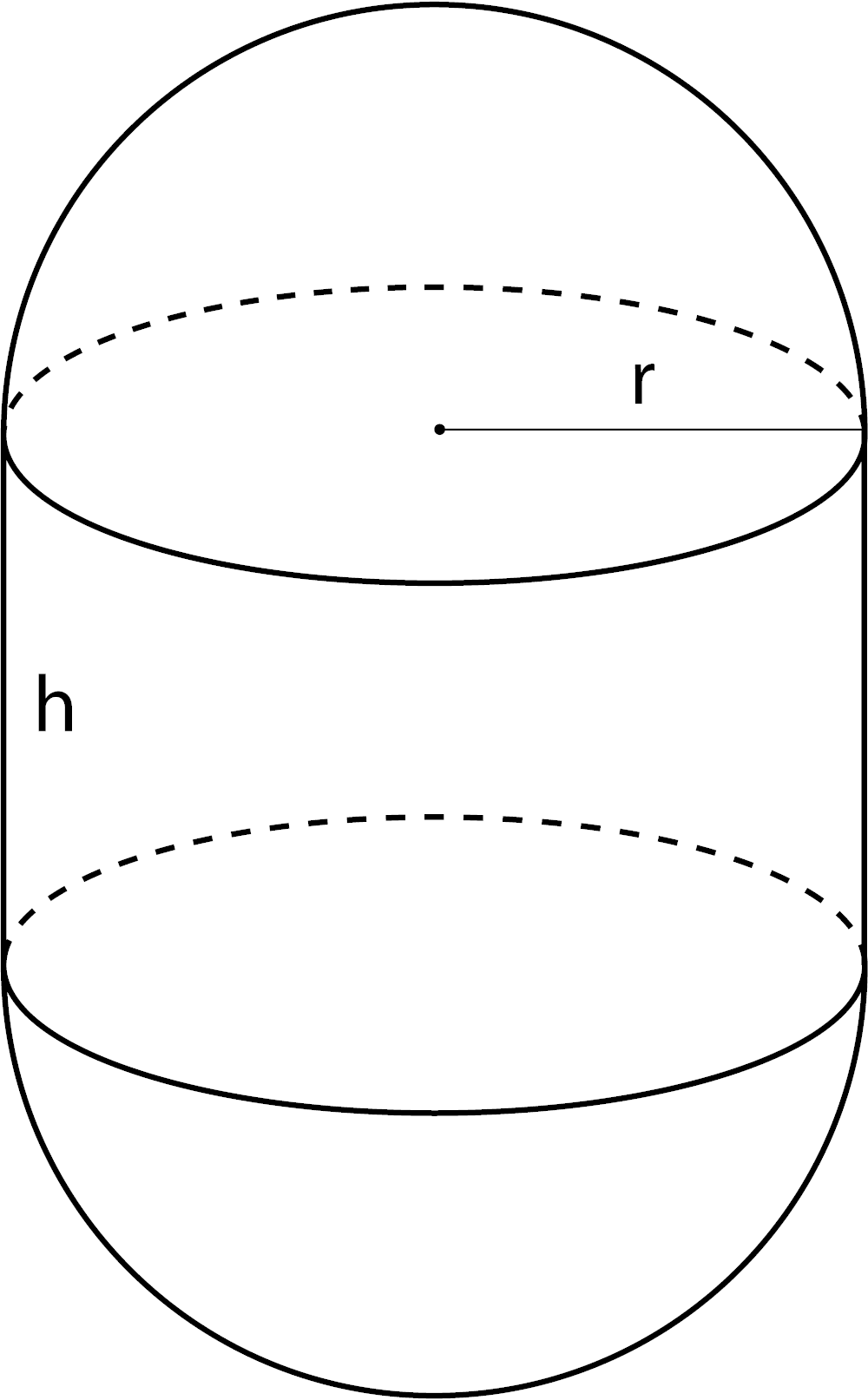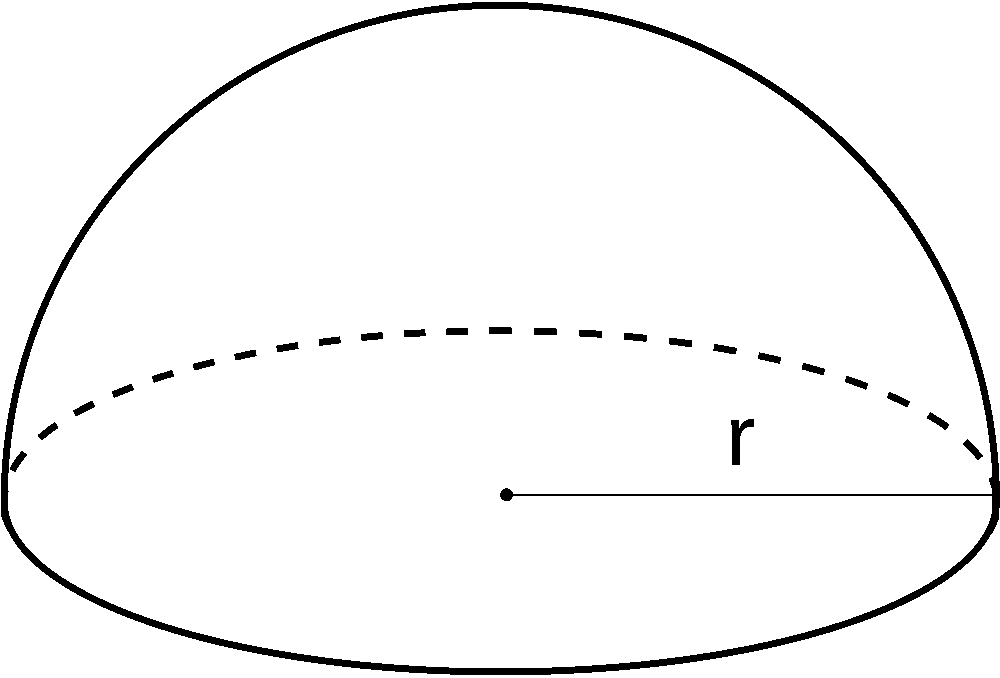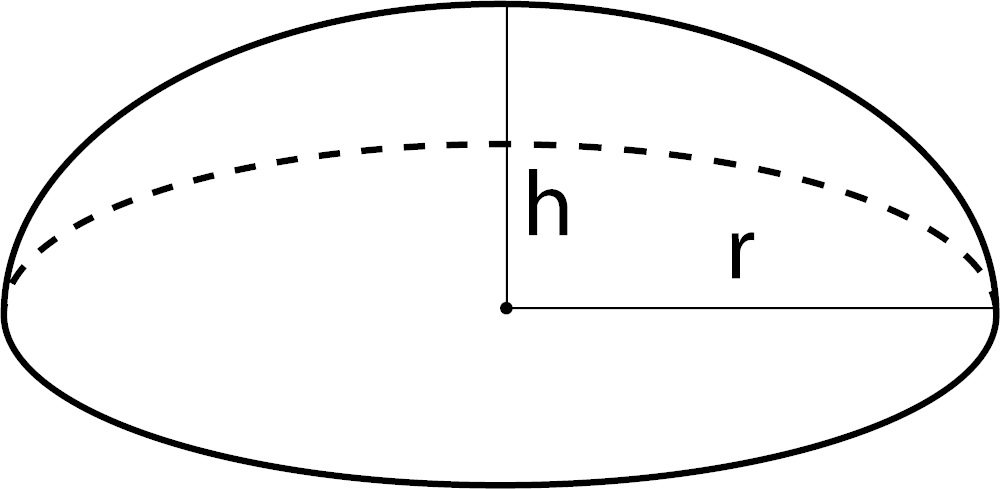## Surface Area:

Learn how we calculated this below

## How to Find Surface Area

Surface area SA is the measure of the area of the surface of a 3-dimensional geometric shape or solid object. Surface area is measured in square units, such as square feet or square meters.

Surface area is equal to the area of each side of an object added together. For instance, the surface area of a cube is equal to the area of each of the six sides of the cube added up.

Considering the cube has 6 equal sides, you can multiply the surface area of one side by six, as shown in the image below.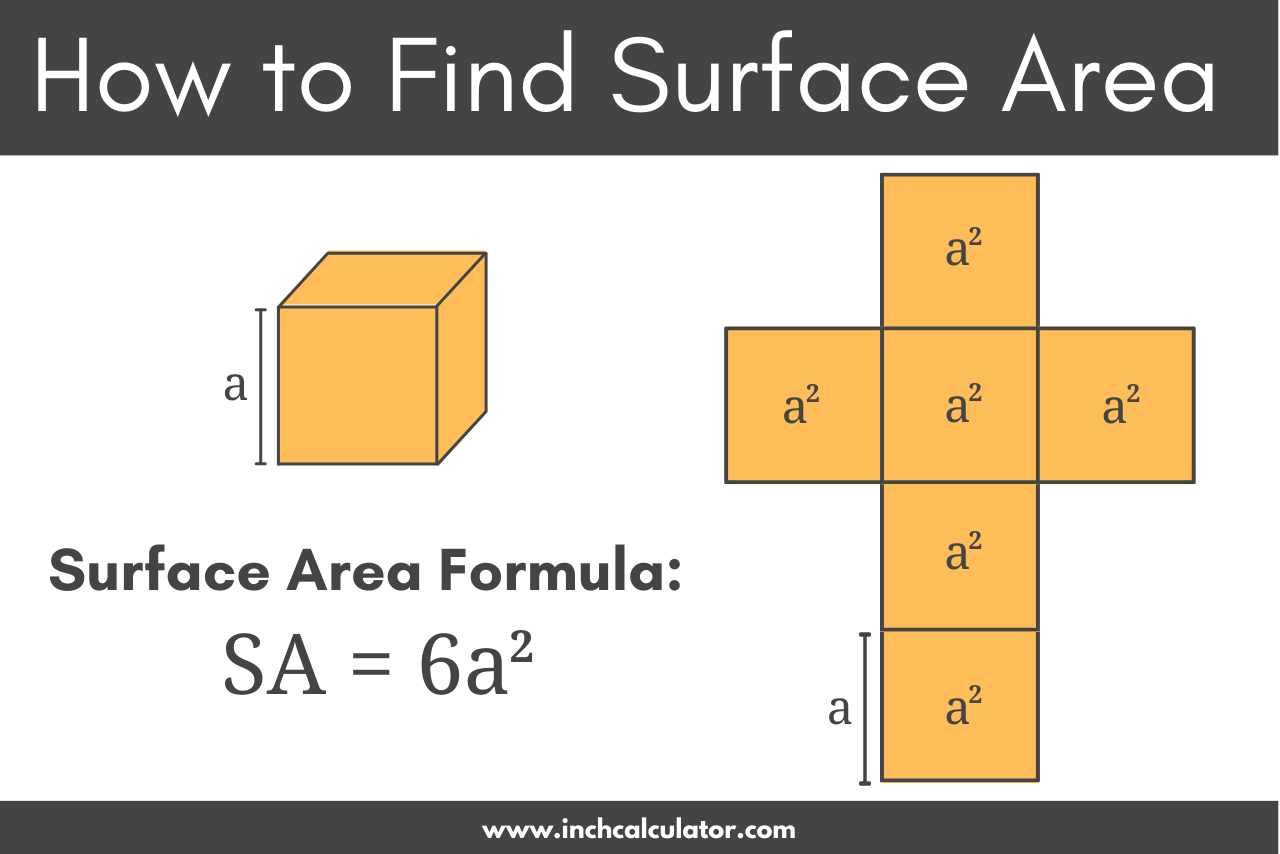There are several types of surface area: curved surface area, lateral surface area, and total surface area.

Curved surface area is the area of the curved regions of an object. An example of a curved region would be the cap area of a hemisphere or capsule.

Lateral surface area is the area the polygon faces of the object, not including the top and bottom bases.

Total surface area is the area of the whole object, including the curved surface, lateral faces, and the area of the base.

When calculating surface area, taking all measurements using the same unit of measure, such as inches or feet, is important. If your measurements are in different units, convert each measurement to the same unit first, then use the formulas below to solve.

The calculator above can easily find the surface area for a shape, but you can also find it using a formula.

## Surface Area Formulas

There is a different surface area formula for each shape. The formula for each shape is below. Use the image as a guide for understanding the formula.

### Surface Area of a Cube

SA = 6a2

a = edge a length### Surface Area of a Rectangular Prism

SA = 2lw + 2lh + 2wh

l = length
w = width
h = height### Surface Area of a Sphere

SA = 4πr2### Surface Area of a Cylinder

SA = 2πr(r + h)

Base SA = 2πr2
Lateral SA = 2πrh

h = height### Surface Area of a Cone

SA = πr(r + r2 + h2)

Base SA = πr2
Lateral SA = πrr2 + h2

h = height### Surface Area of a Pyramid

SA = e2 + 2e(e ÷ 2)2 + h2

Base SA = e2
Lateral SA = 2e(e ÷ 2)2 + h2

e = edge length
h = height### Surface Area of a Triangular Prism

s = 1/2(a + b + c)

Base SA = s(s – a)(s – b)(s – c))
Lateral SA = h × (a + b + c)

You can also use our triangle calculator to find the base surface area using several different methods. This tool is helpful when all sides are not known.### Surface Area of a Capsule

SA = 2πr(2r + h)

Curved SA = 4πr2
Lateral SA = 2πrh

h = height### Surface Area of a Hemisphere

SA = 3πr2

Base SA = πr2
Curved SA = 2πr2### Surface Area of a Spherical Cap

SA = π(r2 + h2) + πr2

Base SA = πr2
Curved SA = π(r2 + h2)

h = height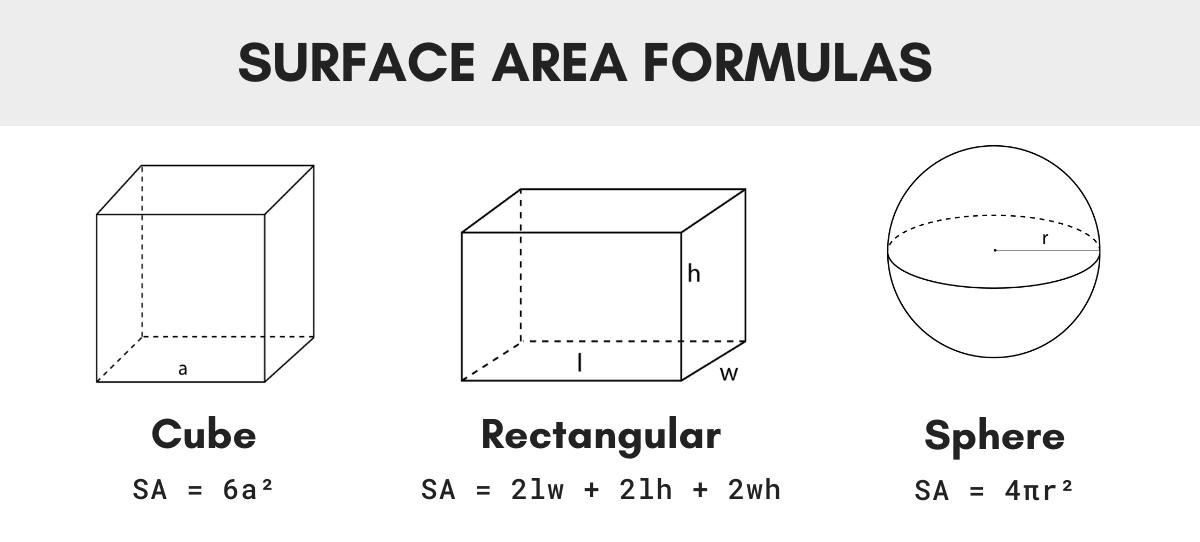You’ll probably also find our volume calculator useful.

### Does surface area mean total area?

The surface area is the total area of the surface of a three-dimensional shape.

### Does surface area mean volume?

Surface area and volume are two different characteristics or attributes of a three dimensional figure. It is possible for two figures to have volumes that are the same but their surface areas are not equal to one another.

### Why is surface area squared?

Surface area is measuring flat surfaces of a figure and then the area surfaces are combined. Since we are finding the area of flat surfaces, the answer is in square units.

We are not finding the measure of the inside of the figure, that would be volume, which would give an answer in cube units.

### Can surface area be cubed?

No. Surface area measures flat surfaces. Therefore, the answer is squared.